# RS Aggarwal Solutions For Class 7 Maths Exercise 2C Chapter 2 Fractions

The pdf of RS Aggarwal Solutions for the Exercise 2C of Class 7 Maths Chapter 2, Fractions is the best study material for those students who are finding difficulties in solving problems. These solutions can help students clear doubts quickly and help in understanding the topic effectively. Our expert tutors formulate these exercises to assist you with your exam preparation to attain good marks in Maths. Students who wish to score good marks in Maths practise RS Aggarwal Class 7 Solutions.

## Download the PDF of RS Aggarwal Solutions For Class 7 Maths Chapter 2 Fractions – Exercise 2C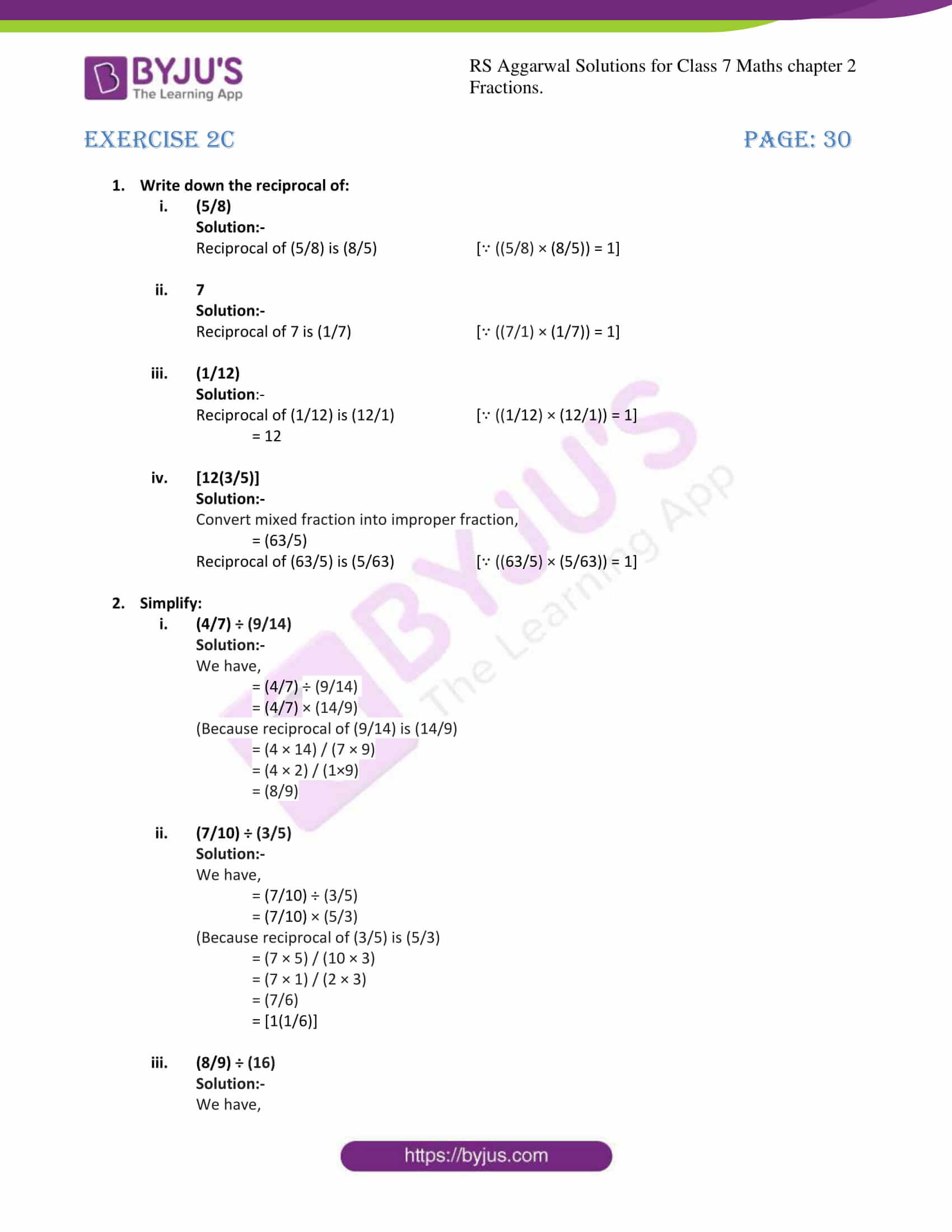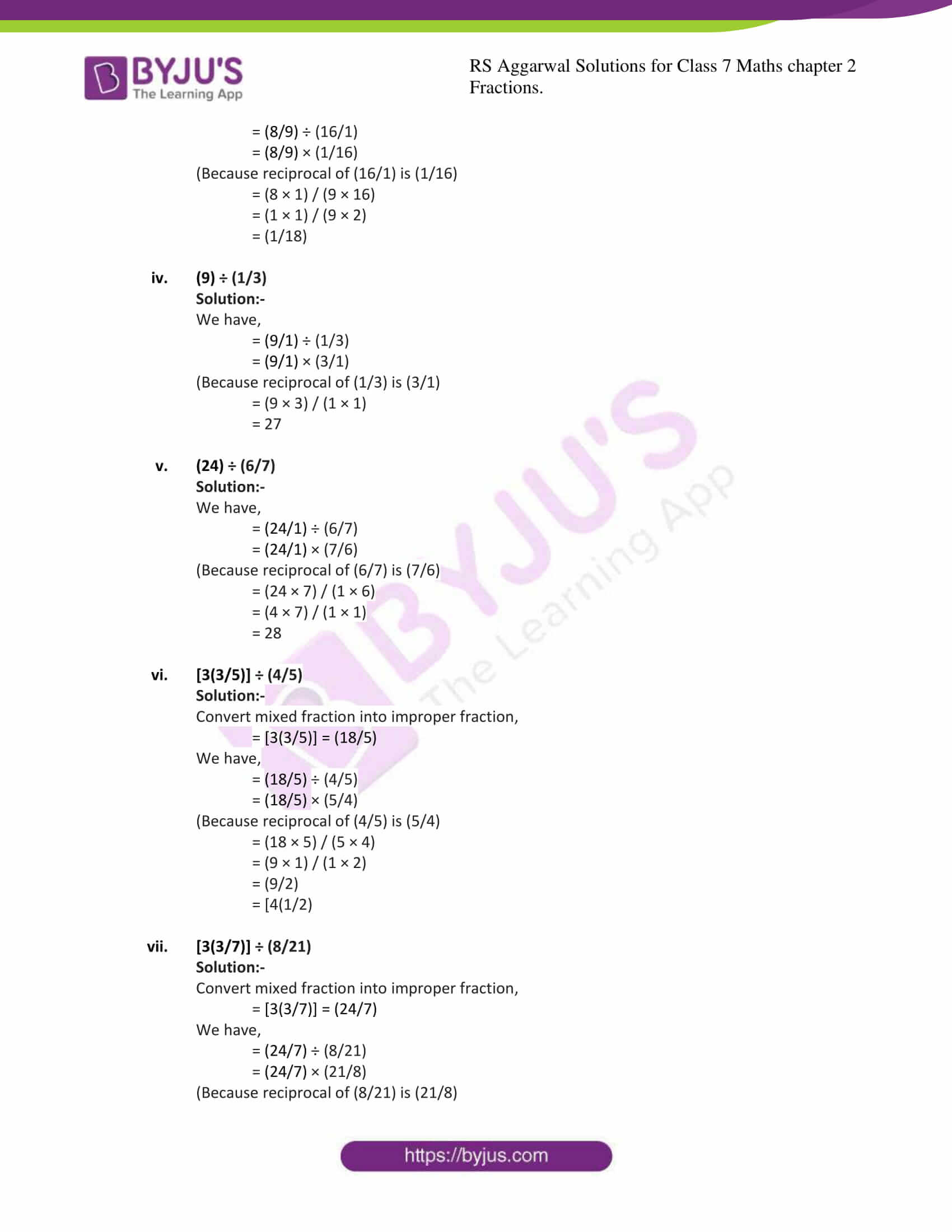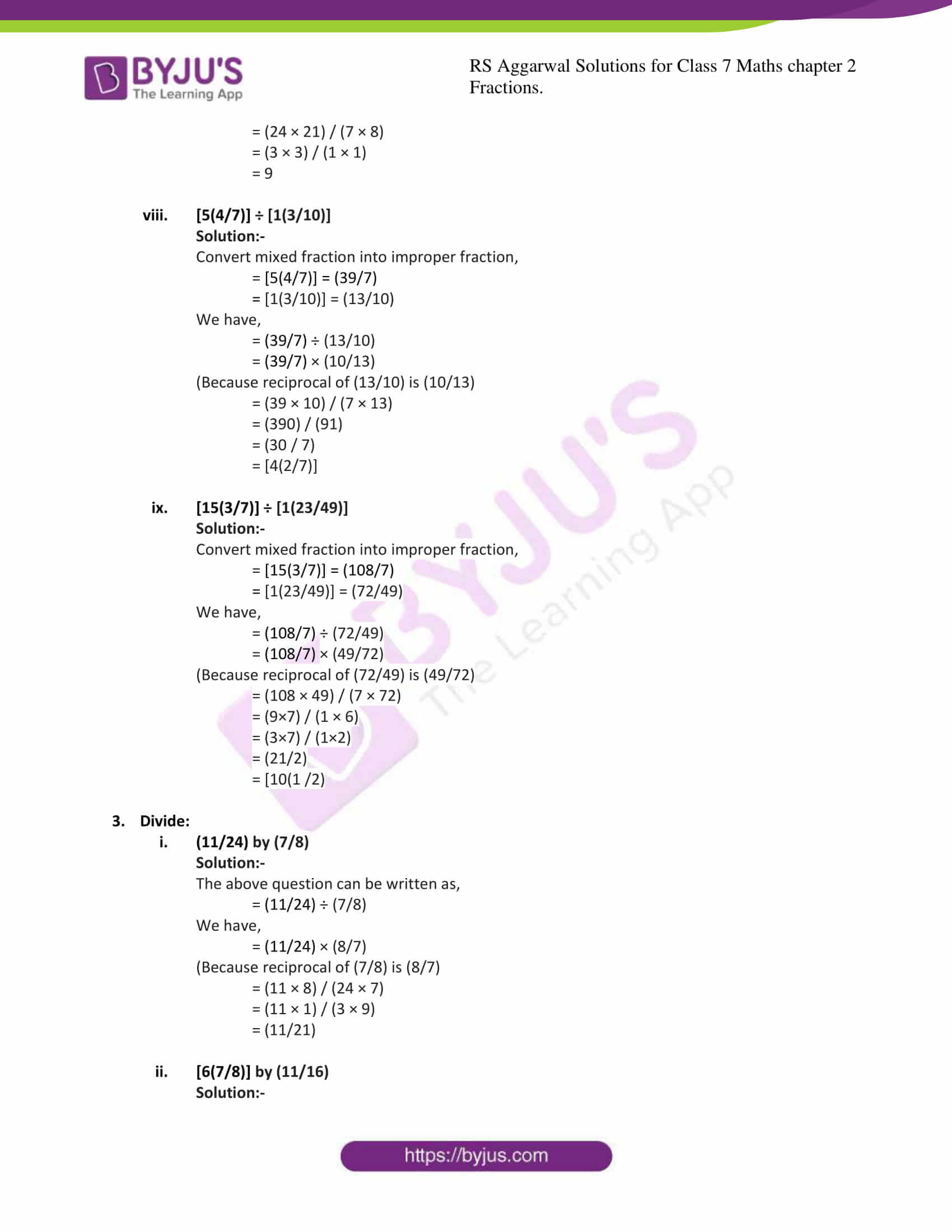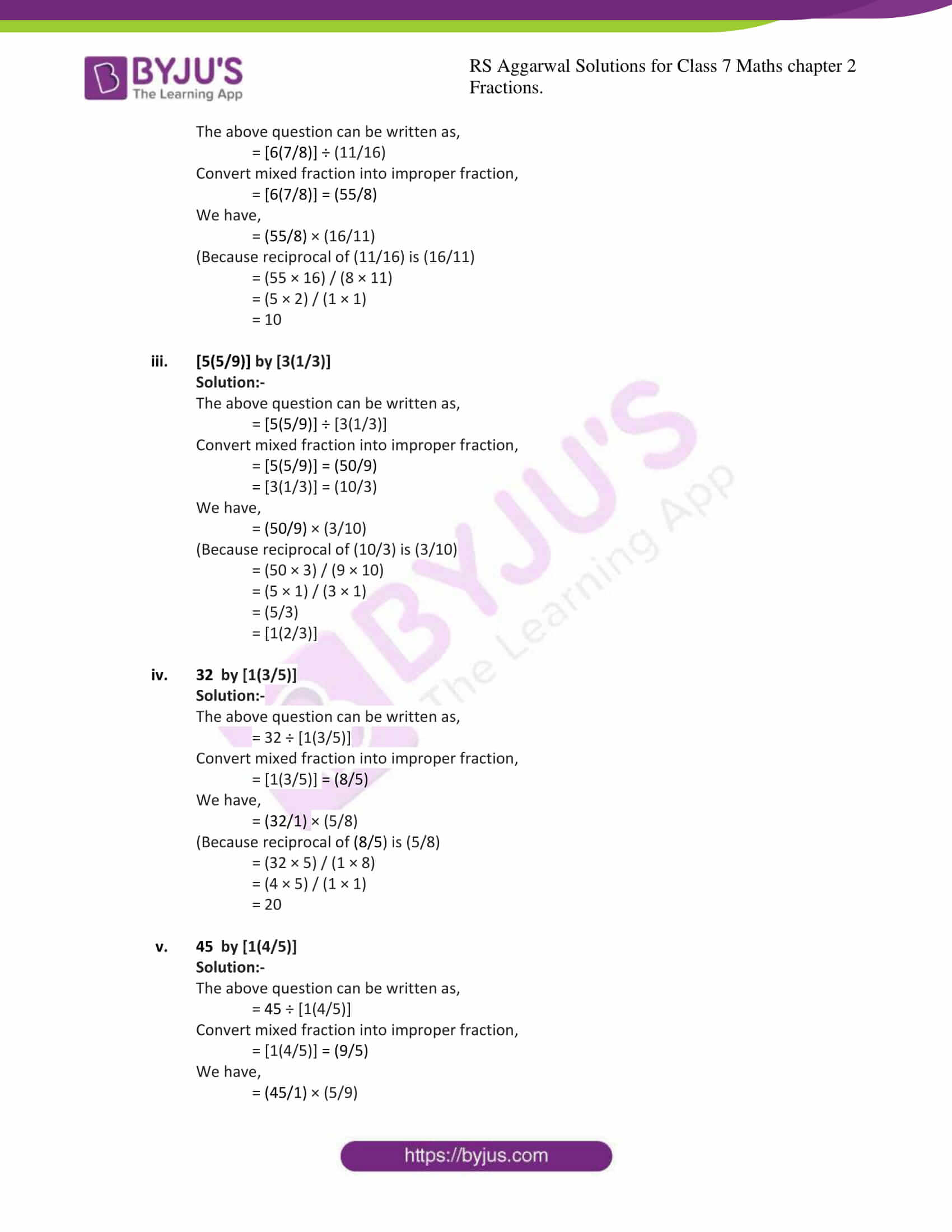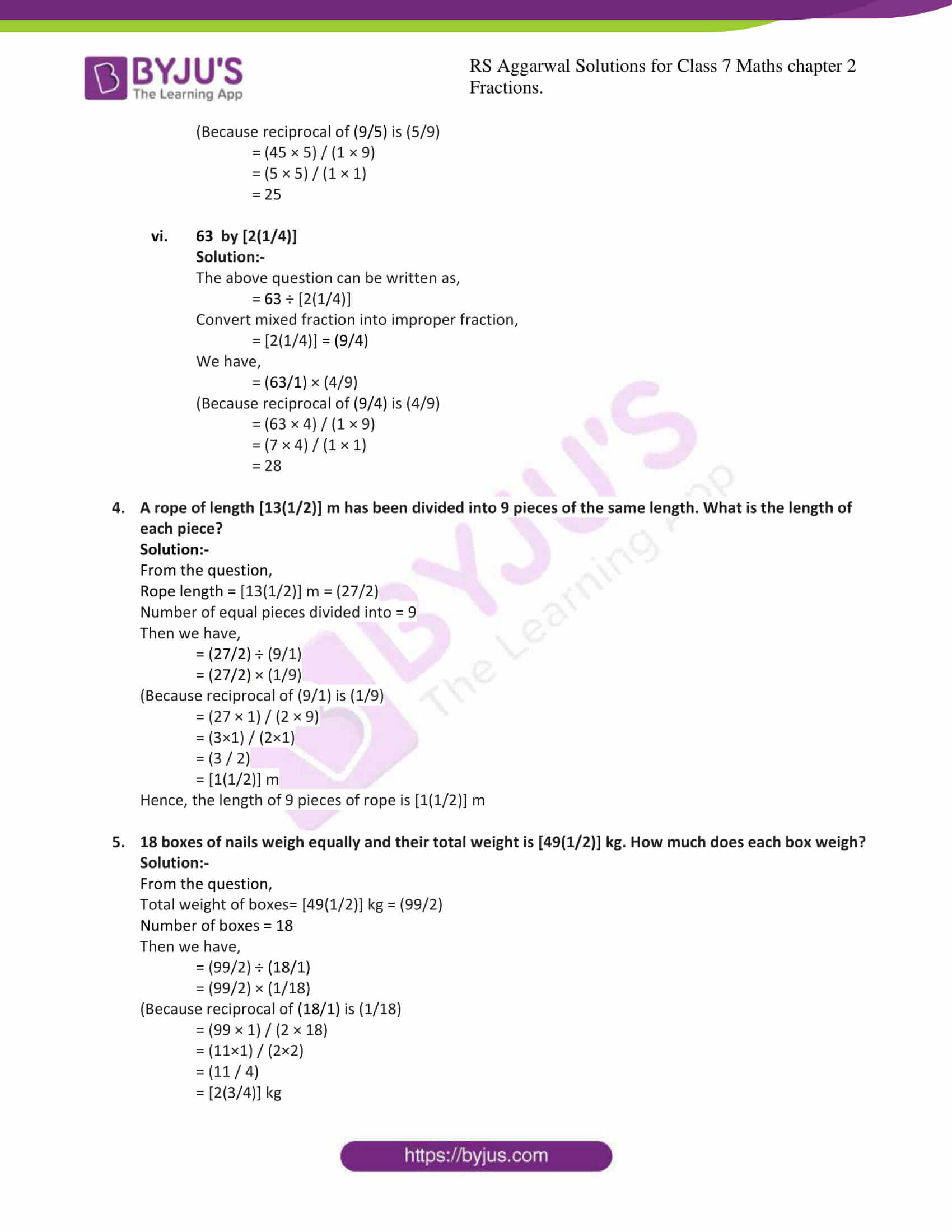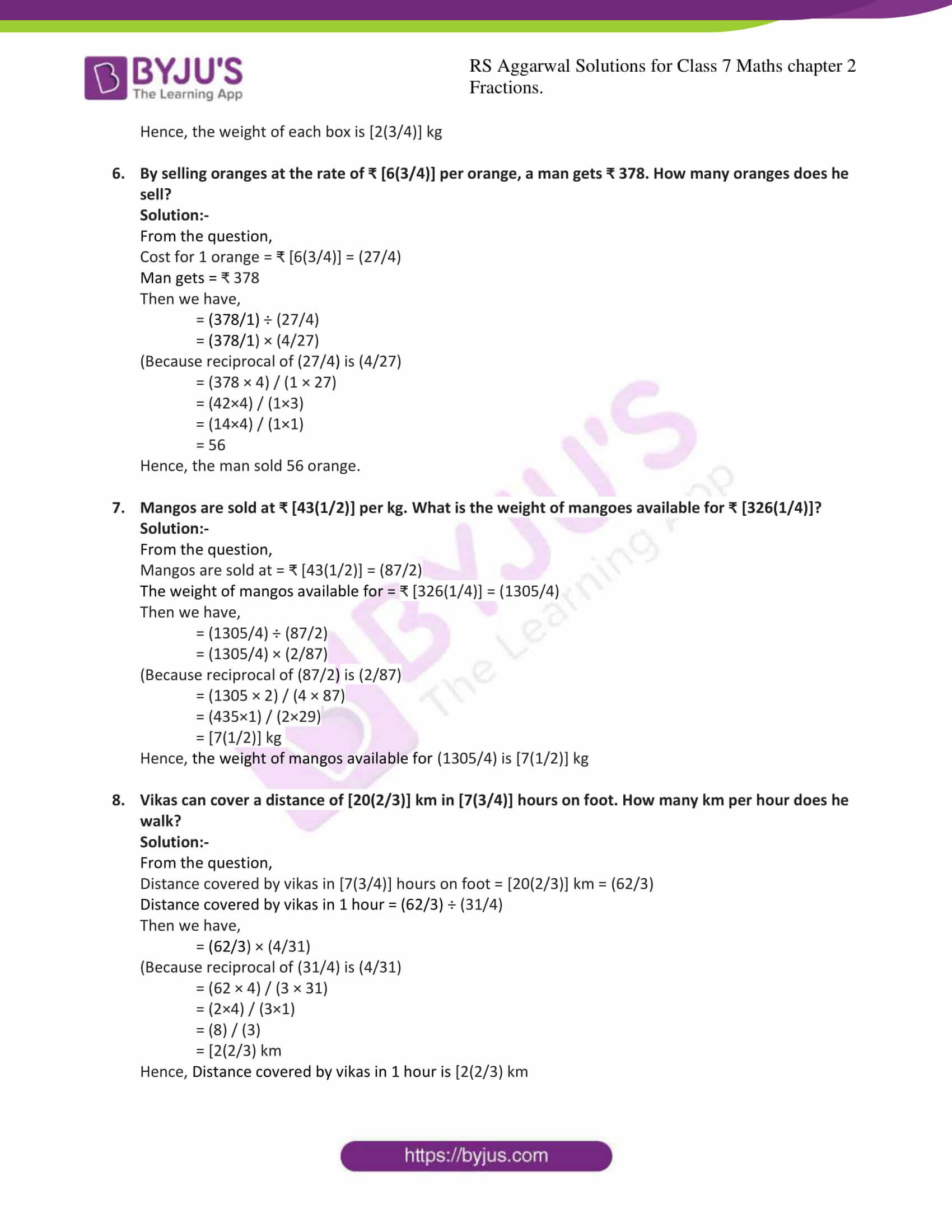### Access answers to Maths RS Aggarwal Solutions for Class 7 Chapter 2 – Fractions Exercise 2C

1. Write down the reciprocal of:
(i) (5/8)

Solution:-

Reciprocal of (5/8) is (8/5) [∵ ((5/8) × (8/5)) = 1]

(ii) 7

Solution:-

Reciprocal of 7 is (1/7) [∵ ((7/1) × (1/7)) = 1]

(iii) (1/12)

Solution:-

Reciprocal of (1/12) is (12/1) [∵ ((1/12) × (12/1)) = 1]

= 12

(iv) [12(3/5)]

Solution:-

Convert mixed fraction into improper fraction,

= (63/5)

Reciprocal of (63/5) is (5/63) [∵ ((63/5) × (5/63)) = 1]

2. Simplify:
(i) (4/7) ÷ (9/14)

Solution:-

We have,

= (4/7) ÷ (9/14)

= (4/7) × (14/9)

(Because reciprocal of (9/14) is (14/9)

= (4 × 14) / (7 × 9)

= (4 × 2) / (1×9)

= (8/9)

(ii) (7/10) ÷ (3/5)

Solution:-

We have,

= (7/10) ÷ (3/5)

= (7/10) × (5/3)

(Because reciprocal of (3/5) is (5/3)

= (7 × 5) / (10 × 3)

= (7 × 1) / (2 × 3)

= (7/6)

= [1(1/6)]

(iii) (8/9) ÷ (16)

Solution:-

We have,

= (8/9) ÷ (16/1)

= (8/9) × (1/16)

(Because reciprocal of (16/1) is (1/16)

= (8 × 1) / (9 × 16)

= (1 × 1) / (9 × 2)

= (1/18)

(iv) (9) ÷ (1/3)

Solution:-

We have,

= (9/1) ÷ (1/3)

= (9/1) × (3/1)

(Because reciprocal of (1/3) is (3/1)

= (9 × 3) / (1 × 1)

= 27

(v) (24) ÷ (6/7)

Solution:-

We have,

= (24/1) ÷ (6/7)

= (24/1) × (7/6)

(Because reciprocal of (6/7) is (7/6)

= (24 × 7) / (1 × 6)

= (4 × 7) / (1 × 1)

= 28

(vi) [3(3/5)] ÷ (4/5)

Solution:-

Convert mixed fraction into improper fraction,

= [3(3/5)] = (18/5)

We have,

= (18/5) ÷ (4/5)

= (18/5) × (5/4)

(Because reciprocal of (4/5) is (5/4)

= (18 × 5) / (5 × 4)

= (9 × 1) / (1 × 2)

= (9/2)

= [4(1/2)

(vii) [3(3/7)] ÷ (8/21)

Solution:-

Convert mixed fraction into improper fraction,

We have,

(Because reciprocal of (8/21) is (21/8)

= (24 × 21) / (7 × 8)

= (3 × 3) / (1 × 1)

= 9

(viii) [5(4/7)] ÷ [1(3/10)]

Solution:-

Convert mixed fraction into improper fraction,

= [5(4/7)] = (39/7)

= [1(3/10)] = (13/10)

We have,

= (39/7) ÷ (13/10)

= (39/7) × (10/13)

(Because reciprocal of (13/10) is (10/13)

= (39 × 10) / (7 × 13)

= (390) / (91)

= (30 / 7)

= [4(2/7)]

(ix) [15(3/7)] ÷ [1(23/49)]

Solution:-

Convert mixed fraction into improper fraction,

= [15(3/7)] = (108/7)

= [1(23/49)] = (72/49)

We have,

= (108/7) ÷ (72/49)

= (108/7) × (49/72)

(Because reciprocal of (72/49) is (49/72)

= (108 × 49) / (7 × 72)

= (9×7) / (1 × 6)

= (3×7) / (1×2)

= (21/2)

= [10(1 /2)

3. Divide:
(i) (11/24) by (7/8)

Solution:-

The above question can be written as,

= (11/24) ÷ (7/8)

We have,

= (11/24) × (8/7)

(Because reciprocal of (7/8) is (8/7)

= (11 × 8) / (24 × 7)

= (11 × 1) / (3 × 9)

= (11/21)

(ii) [6(7/8)] by (11/16)

Solution:-

The above question can be written as,

= [6(7/8)] ÷ (11/16)

Convert mixed fraction into improper fraction,

= [6(7/8)] = (55/8)

We have,

= (55/8) × (16/11)

(Because reciprocal of (11/16) is (16/11)

= (55 × 16) / (8 × 11)

= (5 × 2) / (1 × 1)

= 10

(iii) [5(5/9)] by [3(1/3)]

Solution:-

The above question can be written as,

= [5(5/9)] ÷ [3(1/3)]

Convert mixed fraction into improper fraction,

= [5(5/9)] = (50/9)

= [3(1/3)] = (10/3)

We have,

= (50/9) × (3/10)

(Because reciprocal of (10/3) is (3/10)

= (50 × 3) / (9 × 10)

= (5 × 1) / (3 × 1)

= (5/3)

= [1(2/3)]

(iv) 32 by [1(3/5)]

Solution:-

The above question can be written as,

= 32 ÷ [1(3/5)]

Convert mixed fraction into improper fraction,

= [1(3/5)] = (8/5)

We have,

= (32/1) × (5/8)

(Because reciprocal of (8/5) is (5/8)

= (32 × 5) / (1 × 8)

= (4 × 5) / (1 × 1)

= 20

(v) 45 by [1(4/5)]

Solution:-

The above question can be written as,

= 45 ÷ [1(4/5)]

Convert mixed fraction into improper fraction,

= [1(4/5)] = (9/5)

We have,

= (45/1) × (5/9)

(Because reciprocal of (9/5) is (5/9)

= (45 × 5) / (1 × 9)

= (5 × 5) / (1 × 1)

= 25

(vi) 63 by [2(1/4)]

Solution:-

The above question can be written as,

= 63 ÷ [2(1/4)]

Convert mixed fraction into improper fraction,

= [2(1/4)] = (9/4)

We have,

= (63/1) × (4/9)

(Because reciprocal of (9/4) is (4/9)

= (63 × 4) / (1 × 9)

= (7 × 4) / (1 × 1)

= 28

4. A rope of length [13(1/2)] m has been divided into 9 pieces of the same length. What is the length of each piece?

Solution:-

From the question,

Rope length = [13(1/2)] m = (27/2)

Number of equal pieces divided into = 9

Then we have,

= (27/2) ÷ (9/1)

= (27/2) × (1/9)

(Because reciprocal of (9/1) is (1/9)

= (27 × 1) / (2 × 9)

= (3×1) / (2×1)

= (3 / 2)

= [1(1/2)] m

Hence, the length of 9 pieces of rope is [1(1/2)] m

5. 18 boxes of nails weigh equally and their total weight is [49(1/2)] kg. How much does each box weigh?

Solution:-

From the question,

Total weight of boxes= [49(1/2)] kg = (99/2)

Number of boxes = 18

Then we have,

= (99/2) ÷ (18/1)

= (99/2) × (1/18)

(Because reciprocal of (18/1) is (1/18)

= (99 × 1) / (2 × 18)

= (11×1) / (2×2)

= (11 / 4)

= [2(3/4)] kg

Hence, the weight of each box is [2(3/4)] kg

6. By selling oranges at the rate of ₹ [6(3/4)] per orange, a man gets ₹ 378. How many oranges does he sell?

Solution:-

From the question,

Cost for 1 orange = ₹ [6(3/4)] = (27/4)

Man gets = ₹ 378

Then we have,

= (378/1) ÷ (27/4)

= (378/1) × (4/27)

(Because reciprocal of (27/4) is (4/27)

= (378 × 4) / (1 × 27)

= (42×4) / (1×3)

= (14×4) / (1×1)

= 56

Hence, the man sold 56 orange.

7. Mangos are sold at ₹ [43(1/2)] per kg. What is the weight of mangoes available for ₹ [326(1/4)]?

Solution:-

From the question,

Mangos are sold at = ₹ [43(1/2)] = (87/2)

The weight of mangos available for = ₹ [326(1/4)] = (1305/4)

Then we have,

= (1305/4) ÷ (87/2)

= (1305/4) × (2/87)

(Because reciprocal of (87/2) is (2/87)

= (1305 × 2) / (4 × 87)

= (435×1) / (2×29)

= [7(1/2)] kg

Hence, the weight of mangos available for (1305/4) is [7(1/2)] kg

8. Vikas can cover a distance of [20(2/3)] km in [7(3/4)] hours on foot. How many km per hour does he walk?

Solution:-

From the question,

Distance covered by vikas in [7(3/4)] hours on foot = [20(2/3)] km = (62/3)

Distance covered by vikas in 1 hour = (62/3) ÷ (31/4)

Then we have,

= (62/3) × (4/31)

(Because reciprocal of (31/4) is (4/31)

= (62 × 4) / (3 × 31)

= (2×4) / (3×1)

= (8) / (3)

= [2(2/3) km

Hence, Distance covered by vikas in 1 hour is [2(2/3) km

### RS Aggarwal Solutions for Class 7 Maths Exercise 2C Chapter – 2 Fractions

RS Aggarwal Solutions For Class 7 Maths Chapter 2 Fractions Exercise 2C has problems which are based on the division of fractions. This exercise contains reciprocal of fractions, there are so many sub-questions given to make students solve more along with word problems. Students are suggested to try solving the questions from RS Aggarwal Solutions book of Class 7.